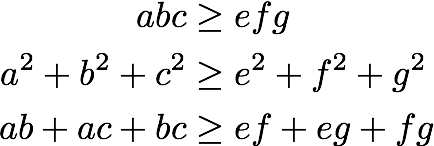One of the many irritating things about airlines is the fact that the cary-on bag restrictions are often stated as “your maximum combined linear measurement (length + width + height) must not exceed 45 inches” when they really mean your bag must fit into a 14 inch by 9 inch by 22 inch box (so they actually may not accept a 43 inch by one inch by one inch pool spear as your carry-on). The “total linear measure” seems (at first glance) “gameable,” but can (through some hairy math) at least be seen to at least be self-consistent. It turns out you can’t put a box with longer total linear measurements into a box with smaller total linear measurements.

Let’s work out why this could be problem and then why the measure works.

The reason we worry about total linear length as a containment measure is a the following related problem. Click and clack (and many others) once shared a puzzle where a boy couldn’t take a five foot fishing rod onto a bus because only objects shorter than four feet are allowed on the bus. The solution is the boy returns with the fishing rod inside a 3 foot by 3 foot by 3 foot box. The trick is this box is considered “shorter than four feet” yet the long diagonal inside the box is over five feet long. The whole puzzle depends on the fact that the longest side of a box is not the longest distance across a box and also not a good measure of box size. So “longest side” of box is a gameable measure; you can put a larger box inside a smaller box if your notion of size is just the longest side of the box.

At first glance it would seem like the sum of the lengths of sides of a box is also not a good measure, as it isn’t related to anything as substantial as volume. Let’s put off working with total linear length for a bit and work with some other measures.

The obvious quantity well related to containment is volume. You can’t put a 10 square foot box inside a 9 square foot box.

Suppose we have two rectilinear boxes. The first box has side of length a,b,c and the second has sides of length e,f,g. We claim that the second box can be placed inside the first. As we have said: there must be enough volume in the first box to hold all of the volume of the second. So we must have a*b*c ≥ e*f*g. We also must have that the long diagonal of the first box (the longest interval in the first box) must be at least as long as the long diagonal of the second box. So we must have sqrt(a*a + b*b + c*c) ≥ sqrt(e*e + f*f + g*g). Surprisingly we must also have that the surface area of the first box is at least as large as the surface area of the second box. So we must have 2*(a*b + a*c + b*c) ≥ 2*(e*f + e*g + f*g).

This surface area fact follows from Cauchy’s surface area formula. Cauchy’s surface area formula implies for bounded convex sets (and both boxes are bounded convex sets) the surface area is proportional expected size of the projected shadow of the set under projections chosen in a uniform radial manner. If you have one bounded convex item contained in another all the projections are simlarly contained and therefore the surface area of the containing box must be at least as large as the contained box. (An even more mathy way to get this fact is: surface area is one of the quermassintegrals, and they are all monotone. You could also try things like mean width and Hadwiger’s theorem.) Anyway, the conclusion is: you need at least as much paint to paint a tent covering a convex building as to paint the building. For convex sets you can’t save surface area by adding on.

Let’s use a little algebra and re-state what we know about our boxes from the fact the second box can be placed in the first:It turns out this enough to already tell us the relation between a+b+c and e+f+g:The last line is substituting the second two facts we know (it turns out we don’t need to use the volume fact). So we know a+b+c ≥ e+f+g (as we know ahead of time both of them should be the positive branch of the square root). Thus the outer box can not be “smaller” in total linear measure. As dumb as it is you can’t game total linear measure, it is at least consistent.

For more on the subtle math of valuations I recommend Klain and Rota’s excellent “Introduction to Geometric Probability.”Found a much quicker treatment of the problem using mixed volumes:
Seven Puzzles You Think You Must Not Have Heard Correctly.

Categories: Expository Writing Mathematics### jmount

Data Scientist and trainer at Win Vector LLC. One of the authors of Practical Data Science with R.